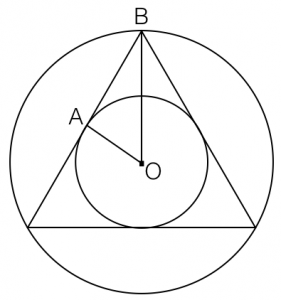Over Age 30 and Taking the GRE? Learn These 3 Key Things!   Get the FREE Video!

# Interpreting “Inscribed” on GRE Quant Questions

GRE geometry questions can be hard for a number of reasons. Obviously the math itself can be difficult, depending on the question. But sometimes the biggest challenge is knowing what a particular word means and/or what a question is even asking.

We talk a lot about beefing up your vocabulary for the GRE verbal section. But in addition to the myriad “big” words you should study for GRE Sentence Completion questions, it’s also worth making sure you know the definition of certain key GRE math words as well.

Inscribed is one of those words — a nuanced math term you’ll likely see on GRE geometry questions. So let’s dissect it and help you get more right answers on test day.

### Understanding the Word Inscribed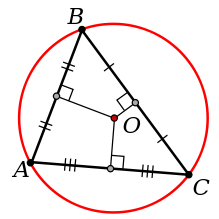Figure 1: A triangle inscribed inside of a circle

The textbook definition of inscribe is as follows: To draw or delineate (one figure) within another figure so that the inner lies entirely within the boundary of the other, touching it at as many points as possible.

Wikipedia describes it as one shape “fitting snugly” inside another geometric shape.

That’s all well and good, but how does that apply to GRE geometry questions? The important understanding is that yes, it’s when one figure is drawn inside another figure, but here’s the really important part: each of the inscribed figure’s vertices much touch the figure it’s inside of. You see that illustrated in Figure 1, where each of the triangle’s vertices, A, B, and C, touches the circumference of Circle O.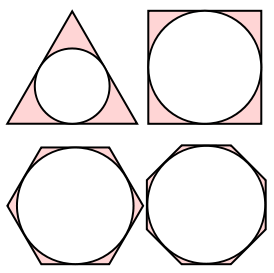Figure 2: Inscribed circles of various polygons

Alternatively, you may see situations where it’s a circle inscribed inside a polygon, as in Figure 2. In that case, since a circle has an infinite number of vertices, the understanding is that the circle touches the perimeter of the polygon it’s inscribed in at as many points as possible. A circle inscribed inside a polygon is tangent to every side of the outer figure, meaning that it touches at exactly one point on each of the faces of the polygon. (There are a few other implicit rules of figures tangent to a circle that you should learn, all of which we cover in more detail in our full GRE math prep course).

One final definition before we look at an application example. To say that “Figure A is inscribed in Figure B” means exactly the same thing as to say that “Figure B is circumscribed about Figure A.” You may see the term circumscribed on the GRE quant section, and now you know what it means.

### Application: Sample GRE Geometry Question

With all of that in mind, consider the following example (below). It was passed along to me by a student who was stumped by how one shape could be inscribed inside another shape of the same kind. Think about the definition provided above and give this question your best shot.

Question: The perimeter of square S is 40. Square T is inscribed in square S. What is the least possible area of square T?

(A) 45
(B) 48
(C) 49
(D) 50
(E) 52

So, how did you do?

Let’s get the suspense out of the way. The correct answer is D, 50. Well done if you got that. Whether you got it right or wrong, let’s take a closer look at a few aspects of the problem that will help you with future GRE math questions as well.

As with all GRE geometry questions for which a figure is not provided, it’s crucial that you use your scratch paper and draw what’s going on in the problem. (Tip: always draw your figures to scale as much as possible). As I always tell my students: he or she who uses the most scratch paper on the GRE, wins.

Drawing Square S is pretty easy. If the perimeter is 40, then each of the sides must be 10 based on the rules of a square, as illustrated here: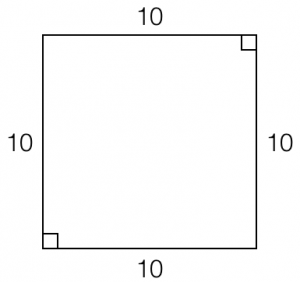Figure 3: Square S, perimeter = 40

The challenging part is knowing how to draw the inscribed Square T. And what do we make of the part of the question that asks for the least possible area of Square T? Is it even possible that Square T could be multiple different sizes? My student went with answer choice C because his thinking was that 49 is the smallest of the answer choices that is a perfect multiple of two integers: 7 * 7 = 49 (remember that the area of a square is one of the sides squared). Of course, the sides of a square don’t have to be integers. It’s possible that the sides of Square T could be √45, in which case √45 * √45 = 45, which is answer choice A.

Or could they?

When you start experimenting with how to draw Square T, you quickly realize that the answer is “no,” there aren’t a lot of hypothetical lengths of the sides of Square T. In fact there’s only one variation of Square T that fits the definition of being inscribed in Square S. Let’s prove it.

You might start drawing Square T like this: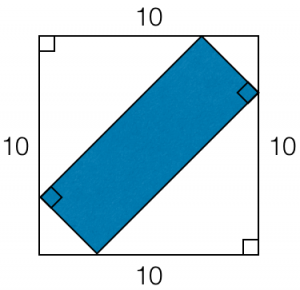Figure 4: Failed attempt at drawing inscribed Square T

But no, that’s clearly more of a rectangle than a square, and quadrilateral T must be a square according to the question. So then maybe you draw Square T like this: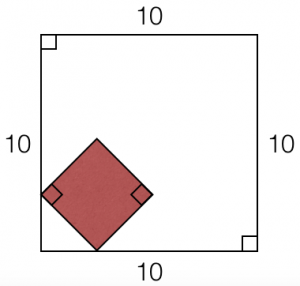Figure 5: Square T inside Square S, but not fully inscribed

That’s good because it’s a pretty small square and the question asks for the least area of square T, after all, but it violates the rules of being inscribed — it only touches at two vertices, whereas it must touch at all four.

So ultimately you realize that Square T must be bigger, “fit snugly” within Square S, and look like this: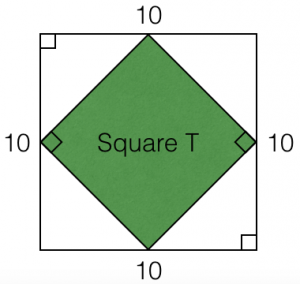Figure 6: Square T fully inscribed inside Square S

Indeed, this is the correct — and only — way of drawing Square T inscribed inside of Square S. The wording in the question is actually redundant; really all it’s asking is for the area of Square T.

So at this point, the math is [hopefully] pretty easy, provided you remember your rules for 45-45-90 right triangles. The area of Square T is the length of one of its sides, squared. Remembering that the hypotenuse of a 45-45-90 right triangle is √2 times as big as its sides, it’s clear that the sides of Square T must be 5√2, as seen here: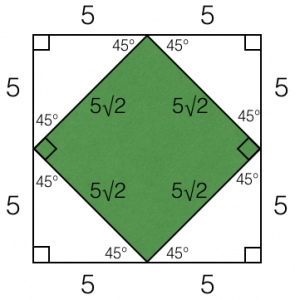Figure 7: Calculating the sides of Square T

The key realization is that because Square T is perfectly inscribed inside Square S, each of its vertices must bisect the sides of Square S. That’s why I’ve labeled Figure 7 as I have, with the full length 10 replaced by two segments of length 5. Hopefully that enables you to see that each of the white corner 45-45-90 right triangles that are created have sides of length 5, and their hypotenuses of length5√2 are correspondingly the sides of Square T. Thus, the area of Square T is 5√2 * 5√2 = 25√4 = 25 * 2 = 50. Answer choice D.

All of this isn’t too bad, is it? The key, of course, is fully understanding what the GRE means when it uses the word inscribed on either discrete problem solving or quantitative comparison questions. And now you know.

So prove it.